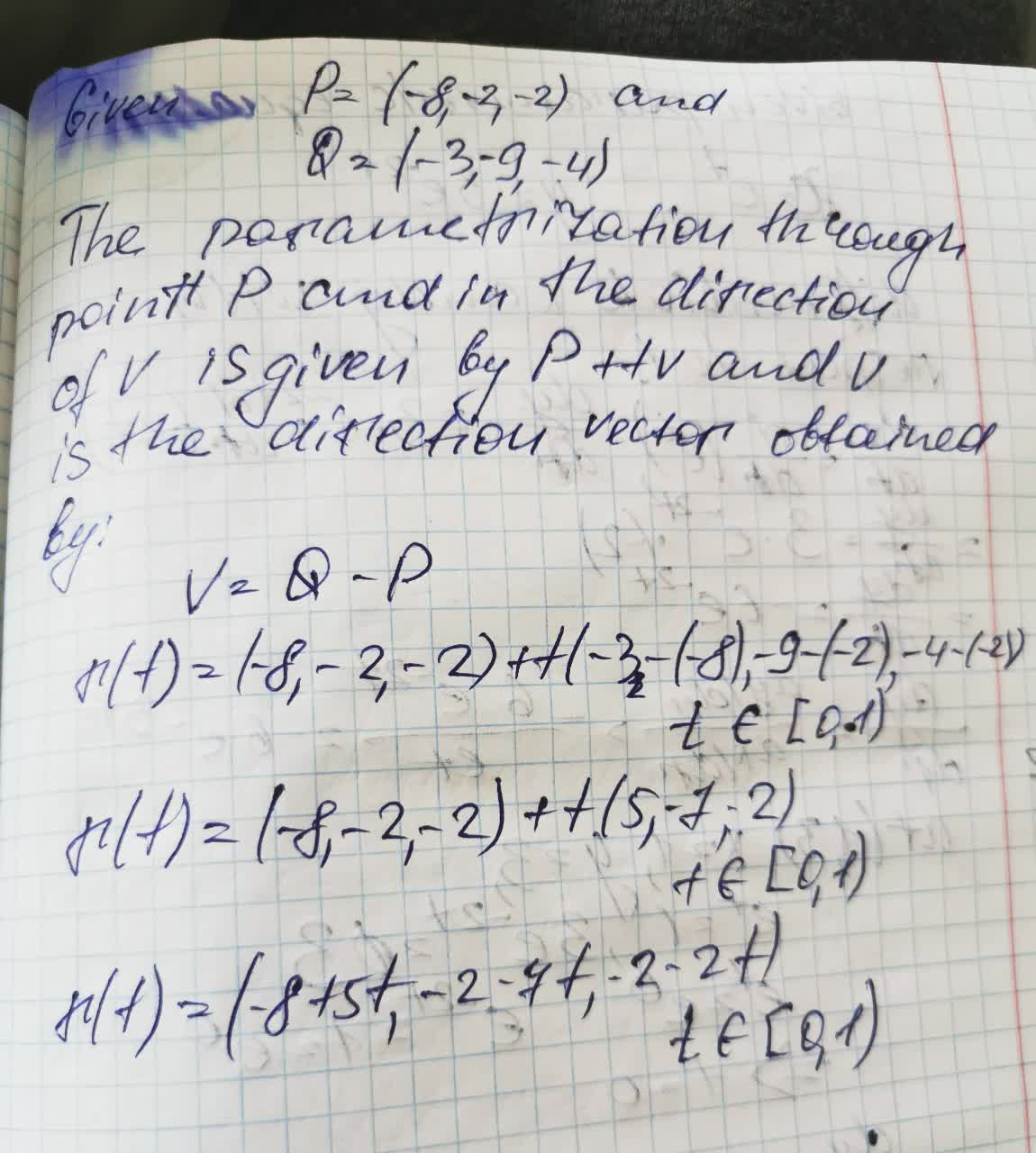Represent the line segment from P to Q by a vector-valued function P(-8,-2,-2), Q(-3,-9,-4)Represent the line segment from P to Q by a set of parametric equationsKaycee Roche 2021-09-06 Answered

Represent the line segment from P to Q by a vector-valued function
$$P(-8,-2,-2), Q(-3,-9,-4)$$
Represent the line segment from P to Q by a set of parametric equations

• Questions are typically answered in as fast as 30 minutes

Solve your problem for the price of one coffee

• Math expert for every subject
• Pay only if we can solve itgrbavit

To find the segment from P to Q by the vector function